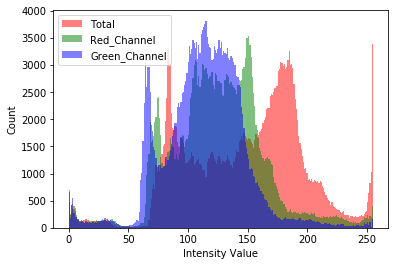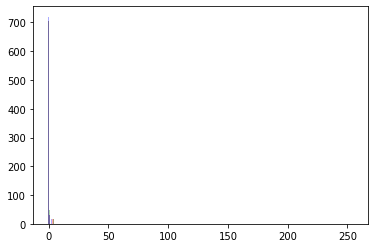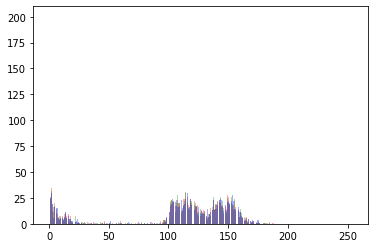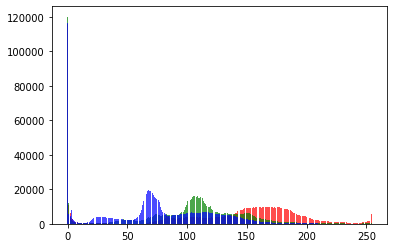# Plot a Histogram for multiple images (full dataset)

Hi, I was able to plot a histogram for a single image as such:

``````from skimage import io
import matplotlib.pyplot as plt

#_ = plt.hist(image.ravel(), bins = 256, color = 'orange', )
_ = plt.hist(image[:, :, 0].ravel(), bins = 256, color = 'red', alpha = 0.5)
_ = plt.hist(image[:, :, 1].ravel(), bins = 256, color = 'Green', alpha = 0.5)
_ = plt.hist(image[:, :, 2].ravel(), bins = 256, color = 'Blue', alpha = 0.5)
_ = plt.xlabel('Intensity Value')
_ = plt.ylabel('Count')
_ = plt.legend(['Total', 'Red_Channel', 'Green_Channel', 'Blue_Channel'])
plt.show()
``````Now, I would know like to see the color distribution of my whole datatset: one plot for all the images.

Does anyone know how to do this?

I assume you cannot load all images into your RAM, so you could instead calculate the bin count for each image and sum it together:

``````nb_bins = 256
count_r = np.zeros(nb_bins)
count_g = np.zeros(nb_bins)
count_b = np.zeros(nb_bins)
for image in range(10):
x = np.random.randint(0, 256, (3, 244, 244))
hist_r = np.histogram(x, bins=nb_bins, range=[0, 255])
hist_g = np.histogram(x, bins=nb_bins, range=[0, 255])
hist_b = np.histogram(x, bins=nb_bins, range=[0, 255])
count_r += hist_r
count_g += hist_g
count_b += hist_b

bins = hist_r
fig = plt.figure()
plt.bar(bins[:-1], count_r, color='r', alpha=0.33)
plt.bar(bins[:-1], count_g, color='g', alpha=0.33)
plt.bar(bins[:-1], count_b, color='b', alpha=0.33)
``````

You might a better answer in a `matplotlib` or `numpy` specific discussion board, but the method should work.1 Like

Cool solution! it works but the plot is very different. I’ll check out a matplotlib forum, good idea!Do you get this plot using my example code snippet?
If so, something seems to be wrong.

Yeah, hearing from some `matplotlib` experts would be good and please share the solution here.Hey, yes, here’s what I used:

``````import numpy as np
from skimage import io
import matplotlib.pyplot as plt

nb_bins = 256
count_r = np.zeros(nb_bins)
count_g = np.zeros(nb_bins)
count_b = np.zeros(nb_bins)

root = './'
for image in os.listdir(root):
if image.endswith('.jpeg'):
hist_r = np.histogram(x, bins=nb_bins, range=[0, 255])
hist_g = np.histogram(x, bins=nb_bins, range=[0, 255])
hist_b = np.histogram(x, bins=nb_bins, range=[0, 255])
count_r += hist_r
count_g += hist_g
count_b += hist_b

bins = hist_r
fig = plt.figure()
plt.bar(bins[:-1], count_r, color='r', alpha=0.33)
plt.bar(bins[:-1], count_g, color='g', alpha=0.33)
plt.bar(bins[:-1], count_b, color='b', alpha=0.33)

``````

No! I was comparing two different ones. Here’s for the same image:Would it be possible to upload this image here?

YesI get quite the same results for this image using the “manual” approach and `plt.hist`:

Manual:`plt.hist`:So you used my code for plot one and your code for plot two? That’s odd.

Can you paste the code you used to plot your method by passing an image (and not rand)

Also! if you notice, the y axis values are quite different

Ah, I had an error in the code summing into `count_x` instead of resetting, as I used my code for multiple images. I get the same counts now for both approaches using this code:

``````nb_bins = 256
count_r = np.zeros(nb_bins)
count_g = np.zeros(nb_bins)
count_b = np.zeros(nb_bins)

img = Image.open('./discuss_eye_test.jpeg')

# Calculate manual hist
x = np.array(img)
x = x.transpose(2, 0, 1)
hist_r = np.histogram(x, bins=nb_bins, range=[0, 255])
hist_g = np.histogram(x, bins=nb_bins, range=[0, 255])
hist_b = np.histogram(x, bins=nb_bins, range=[0, 255])
count_r = hist_r
count_g = hist_g
count_b = hist_b

# Plot manual
bins = hist_r
fig = plt.figure()
plt.bar(bins[:-1], count_r, color='r', alpha=0.5)
plt.bar(bins[:-1], count_g, color='g', alpha=0.5)
plt.bar(bins[:-1], count_b, color='b', alpha=0.5)

# Plot matplotlib
fig2 = plt.figure()
plt.hist(x.ravel(), bins = 256, color = 'red', alpha = 0.5)
plt.hist(x.ravel(), bins = 256, color = 'green', alpha = 0.5)
plt.hist(x.ravel(), bins = 256, color = 'blue', alpha = 0.5)
``````

Cool! I have the same result as well.

How can you now compute multiple images? Do you need something like:

``````for image in os.listdir('test_images'):
img = Image.open(image)
x = np.array(img)
``````

That only seems to plot the histo of the last image.

My initial code snippet should accumulate the counts of all images into the `count_x` arrays, so that you could plot the histogram of all images.

Got it, I changed it just a bit:

``````nb_bins = 256
count_r = np.zeros(nb_bins)
count_g = np.zeros(nb_bins)
count_b = np.zeros(nb_bins)

for image in os.listdir('./test/'):
img = Image.open('./test/'+image)
x = np.array(img)
x = x.transpose(2, 0, 1)
hist_r = np.histogram(x, bins=nb_bins, range=[0, 255])
hist_g = np.histogram(x, bins=nb_bins, range=[0, 255])
hist_b = np.histogram(x, bins=nb_bins, range=[0, 255])
count_r += hist_r
count_g += hist_g
count_b += hist_b

bins = hist_r
fig = plt.figure()
plt.bar(bins[:-1], count_r, color='r', alpha=0.7)
plt.bar(bins[:-1], count_g, color='g', alpha=0.7)
plt.bar(bins[:-1], count_b, color='b', alpha=0.7)
``````Any idea why the plotted curves break like that?

1 Like

Hi
sorry , I am trying to have histogram of the tensor which is between 0 to 1. I used this command but it shows me nothing. I check the bins and Counter have numbers but show nothing.

``````        hist_r = np.histogram(kk.squeeze(0).view(-1).detach().numpy(),bins=100)
fig = plt.figure()
bins = hist_r
count_r=hist_r
plt.bar(bins[:-1], count_r, color='b', alpha=0.33)
``````

Is my example code working for you or is the figure also empty?

I redo it again it work. Would you please tell me how I can define the bins in the sequence of the numbers ? like that bins=[0:0.1:1] or [0:10:255]

You can pass the bin edges to the `bins` argument directly in `np.histogram`.
From the docs:

bins int or sequence of scalars or str, optional
If bins is an int, it defines the number of equal-width bins in the given range (10, by default). If bins is a sequence, it defines a monotonically increasing array of bin edges, including the rightmost edge, allowing for non-uniform bin widths.
New in version 1.11.0.
If bins is a string, it defines the method used to calculate the optimal bin width, as defined by `histogram_bin_edges` .

Hi Ptrblck,

Would you please help me with that which function I can use to give me the density function, the smooth version of the histogram? I used the current function but it gives me individual bins, I need a smoothed version.